# Multiplexed Readout of Superconducting Qubits with the UHFQA

January 15, 2020 by Max Ruckriegel

Frequency-multiplexed readout of qubits is at the heart of superconducting quantum processors and an essential step for scalable architectures. The UHFQA Quantum Analyzer is specifically tailored for this challenge and brings the latest technology to work in your lab - using high level API. In this blog post we demonstrate a workflow for reading out ten qubits with all the essential features: matched filter weights, crosstalk suppression, and real-time state discrimination.

## Tabletop Demonstration - Measuring Ten Qubits Without a Lab

To give an introductory overview of the main UHFQA functionalities, we have put together an interactive demonstration that does not rely on any actual superconducting qubits in a cryostat. Here we only need two BNC cables that connect the two signal outputs to the two inputs in a closed loop as shown in Figure 1. We mimic the qubit-state dependent resonator response with a trick: depending on the defined state of a qubit, we add small amplitude and phase shifts to the measurement tone associated to that qubit. In this way, we "fake" the multiplexed readout signals to resemble signals that would be acquired when measuring multi-qubit states.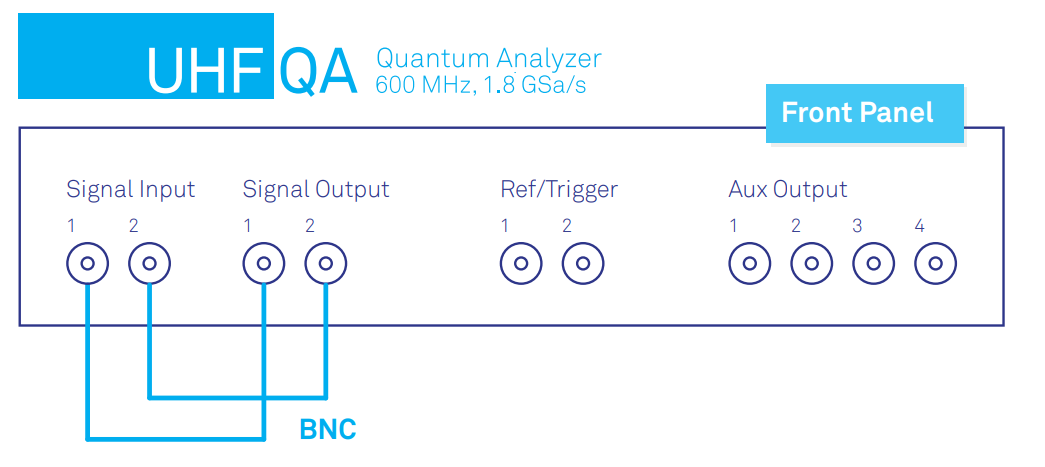Figure 1: The UHFQA Quantum Analyzer with its outputs connected to the inputs in a closed loop for a tabletop demonstration.

In a real-life experiment, the instrument would of course not be connected in a closed loop; the UHFQA would be used to probe a superconducting device in a cryostat. Typically, an IQ-mixer with a local oscillator at around 7 GHz up-converts the readout signal to a ±600 MHz band around the LO frequency. The two signal outputs serve as the in-phase and quadrature components of the readout pulse. A second IQ-mixer with the same LO down-converts the returning signal from the fridge and feeds the two signal inputs of the UHFQA with the I and Q components for analysis.

### Jupyter notebook

The core of this demonstration is a Jupyter notebook that can be found here: https://github.com/zhinst/blogs/Multiplexed Readout of Superconducting Qubits.

We make use of the ziPython API to control the UHFQA interactively from within the notebook. The first steps are:

• Import helper function from multiplexed_readout_helpers.py. It includes functions for creating an AWG sequence program, for initializing device settings or for starting the AWG.
• Establish a connection to the LabOne Data Server and then connect the device.
# connect to LabOne Data Server
device = 'dev2266'
discovery = zi.ziDiscovery()
device_id = discovery.find(device)
device_props = discovery.get(device_id)

device_props['serverport'],
device_props['apilevel'])
daq.connectDevice(device, '1GbE')
• Define parameters for multiplexed readout such as the number of channels used and the corresponding readout frequencies.
# define readout frequencies for 10 channels
frequencies = 1e6 * np.linspace(82.6, 114.7, 10)
n_channels = len(frequencies)
• Generate readout signals with the AWG and record the measurement results. The generated AWG sequence adds a small offset in amplitude and phase to each readout tone, depending on the corresponding entry in the state parameter. We subscribe to the result_paths we want to acquire and then poll the results using the acquisition_poll() function after starting the AWG.
...
# define states
ground_state = 
excited_state = 

# iterate over ground/excited state
for state in [ground_state, excited_state]:
# create sequence program, compile and send to AWG
channels,
frequencies,
result_length*result_averages,
state=state
)
compile_sequence(awg, awg_program)

# sync device, subscribe to path and run AWG
daq.sync()
daq.subscribe(result_path)
run_awg(daq, device)

# poll data from DAQ
result_list.append(acquisition_poll(daq, result_path, result_length))
daq.unsubscribe(result_path)
...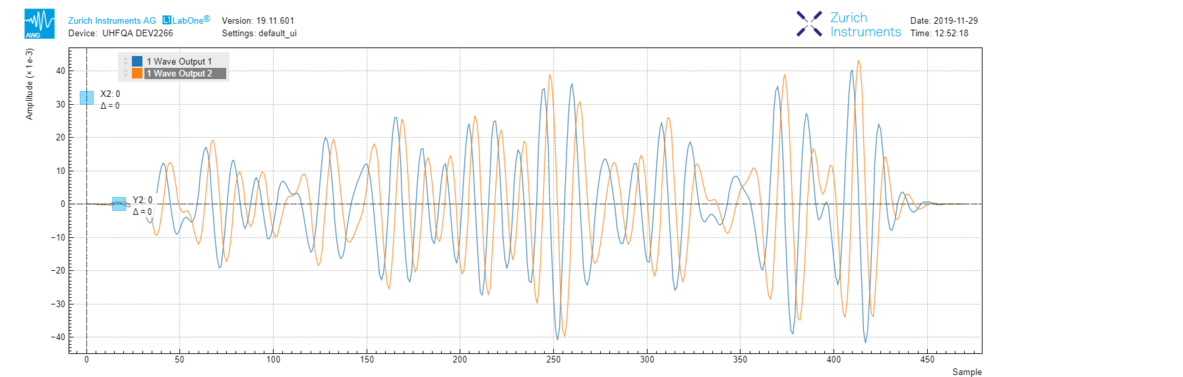Figure 2: Generated AWG waveform (I and Q quadrature) composed of 10 readout tones.

## Weighted Integration with the UHFQA

For simple digital demodulation, it is enough to set the integration weights of a channel to a sine wave at the desired demodulation frequency. Especially for short readout pulses, however, it becomes important to consider the resonator ring-up and properly weight the time-dependent signal for best signal-to-noise ratio.

We employ a simple technique to find the optimal integration weights for our example, see . We measure the I and Q traces of the resonator response twice, once with the qubit in its excited state and once with the qubit in its ground state. The averaged differences

$w_I = \langle V_{I,e} - V_{I,g} \rangle$

$w_Q = \langle V_{Q,e} - V_{Q,g} \rangle$

provide the optimal integration weights for the two quadratures. Conveniently, these differences oscillate with the chosen readout frequency such that the demodulation frequency does not have to be explicitly accounted for.

...
reset_integration_weights(daq, device, channels=[channel])

# path to result data for single channel
monitor_paths = [
f"/{device}/qas/0/monitor/inputs/0/wave",
f"/{device}/qas/0/monitor/inputs/1/wave",
]

ground_state = 
excited_state = 

# iterate over ground and excited state
for state in [ground_state, excited_state]:
#  * generate AWG program
#  * run AWG
#  * data acquisition --> waves

wave_I_0 = waves
wave_Q_0 = waves
wave_I_1 = waves
wave_Q_1 = waves

# simply take difference
weights_I = wave_I_1 - wave_I_0
weights_Q = wave_Q_1 - wave_Q_0

# set new integration weights
set_integration_weights(daq, device, weights_Q, channel, quadrature="imag")

The QA Input Monitor acquires the traces on the two signal inputs for the qubit in the ground or excited state (first and second panel). Signal inputs 1 and 2 correspond to the I and Q quadrature of the readout signal. The two signals differ by a qubit-state dependent amplitude and phase shift. We set the integration weights for both quadratures to the difference between the acquired traces for the ground and excited states which allows us to optimally distinguish between the two states.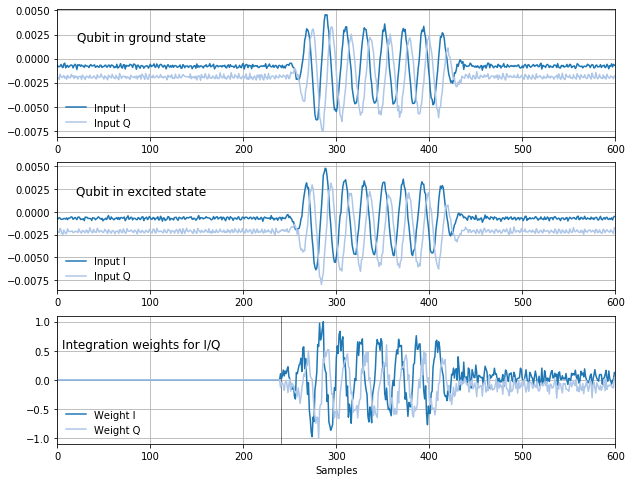Figure 3: I and Q quadratures at the two signal inputs acquired by the QA Input Monitor. The traces are measured with the qubit either in ground or excited state. Their difference is used for weighted signal integration.

After setting the integration weights, the two point clouds in the IQ-plane (ground and excited state) are at ~45° from each other (left plot in Figure 4). This corresponds to maximizing the difference of the two states for both quadratures individually. Because in the next analysis steps the UHFQA works with only real valued signals, we rotate the complex values such that the entire readout signal is in only one quadrature (right plot) and set the QA Rotation angles to the corresponding values.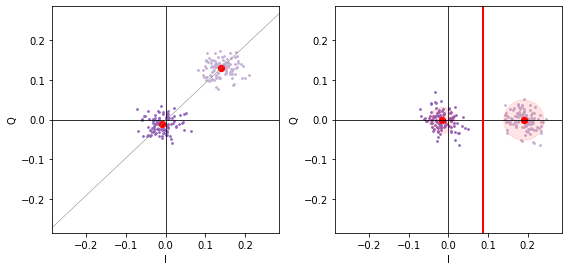Figure 4: Point clouds of excited (light violet) and ground state (dark violet) after the QA integration unit. The signals are rotated by the calculated angle between the averaged signals. Afterwards a threshold in the I quadrature (red line) can be used to discriminate the states on the UHFQA hardware.

For further analysis of the qubit state we will later define a separation threshold (red line) to distinguish the two states depending on the output signal after rotation, i.e., in the I-quadrature.

## Minimizing Measurement Crosstalk

In real-life experiments, the readout signals can be strongly affected by measurement crosstalk that eventually decreases the state assignment fidelity. Luckily, the UHFQA comes with a built-in crosstalk compensation matrix which helps solve this issue. Linear crosstalk is suppressed by choosing appropriate entries in the compensation matrix that diagonalize the present crosstalk after the QA Rotation unit. With proper calibration, a signal change at a single readout frequency should only affect the signal of the associated result channel – leading to higher multiplexed readout fidelities.

Signal processing up to the QA Crosstalk stage is described by the $$10 \times 10$$ matrix $$M$$ that transforms the multi-qubit state $$\left( s_1, s_2, ... , s_{10} \right)$$ with $$s_q=0$$ or $$1$$ to the readout voltages $$\left(V_1, V_2, …, V_{10} \right)$$. We obtain this crosstalk matrix systematically by preparing all the different states of the form $$\left( 0, ..., 1, ... \right)$$ and measuring the resulting signals on all channels. Compensation of crosstalk can be achieved by finding the matrix $$C$$ that diagonalizes $$C \times M$$. This implies that as soon as we precisely know $$M$$ we can always multiply the signals by $$C = M^{-1}$$ to suppress the effect of measurement crosstalk.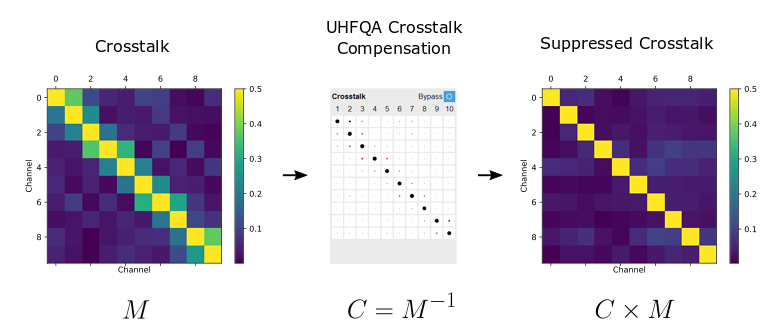Figure 5: Crosstalk calibration with the built-in crosstalk suppression matrix of the UHFQA. The matrix on the left shows considerable crosstalk before calibration. Setting the compensation matrix of the QA to the inverse of $$M$$ (center) significantly reduces measurement crosstalk (right).

We follow a simple calibration procedure to find $$M$$ and eventually set the compensation matrix in the QA Setup tab to its inverse. After making sure the compensation matrix initially is the identity matrix, we consecutively prepare all ten different states with only a single qubit excited while all others are in their ground state.

The measured voltages of each channel are compared to the signals that are measured when all the qubits are in their ground state. The normalized signal change immediately shows how a state change of qubit $$i$$ affects the readout signal of channel $$j$$ and thus quantifies the unwanted crosstalk for channels $$j \neq i$$ . By repeating this measurement for every qubit we assemble the entire crosstalk matrix $$M$$ row by row. Non-zero off-diagonal entries in $$M$$ show the presence of crosstalk.

At $$M$$ in Figure 5 shows that crosstalk is strongest between neighboring channels due to a finite spectral overlap of the readout tones. Having found $$M$$ , we set the entries of the compensation matrix to the inverse $$C = M^{-1}$$ which can be implemented like this thanks to the ziPython API for LabOne:

write_crosstalk_matrix(daq, np.linalg.inv(ct_matrix))
...

# --------------------------------------

def write_crosstalk_matrix(daq, matrix):
rows, cols = matrix.shape
for r in range(rows):
for c in range(cols):
node = f"/{device}/qas/0/crosstalk/rows/{r}/cols/{c}"
daq.setDouble(node, matrix[r, c])
return

The matrix updated in LabOne is shown in the center image in Figure 5. Now that we have calibrated the compensation matrix, we can check its effect on the readout signals. A second measurement of $$M$$ illustrates how well the crosstalk could be suppressed. In our simple example, the compensation matrix reduces the off-diagonal entries of $$M$$ by one order of magnitude.

Note that for this example we have set all ten readout signals to be within a very narrow frequency range with a spacing of only few MHz between two readout tones. The spectral overlap of the individual tones is thus relatively large and the crosstalk is considerable. With the UHFQA, even such a large measurement crosstalk can be reduced to an acceptable level.

## Thresholding for State Discrimination

We can now calibrate the thresholding function of the UHFQA. For each channel, we define a voltage level that sets the threshold between the states $$0$$ and $$1$$ such that the instrument eventually returns a state $$\left( s_1, s_2, ... , s_{10} \right)$$ with $$s_q=0$$ or $$1$$ rather than voltages $$\left(V_1, V_2, …, V_{10} \right)$$. To find the best value for the discrimination threshold we prepare the ground state $$\left( 0, ..., 0 \right)$$ as well as all the states with only a single excited qubit, e.g. $$\left( 1, 0, ..., 0 \right)$$ for channel 1. We then calculate the threshold level as the average of the mean signal levels for the two states, marked as solid and dashed red lines in Figure 6.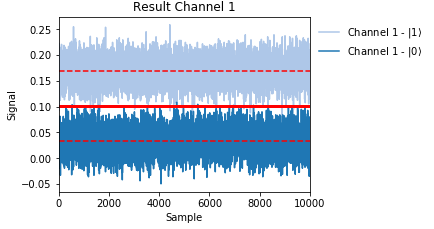Figure 6: Calibrating the signal threshold for state discrimination.

Taking many averages also allows us to determine the probability of assigning the wrong state. In Figure 7 we plot the data as histograms to show the probability distribution for measuring a certain signal above or below the threshold (vertical black line) for excited and ground state (red and blue).

Channel 1:

P(e|g) = 0.0025
P(g|e) = 0.0051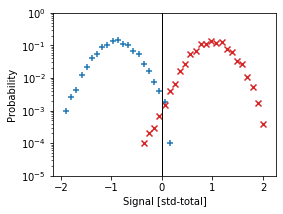Figure 7: Probability distributions of measured signals for the qubit in excited (red) and ground (blue) state.

After setting up the signal threshold for each of the ten channels we set the signal source for the QA Result tab to be the QA Thresholding unit. The measured signal in the QA Result tab is now a multi-qubit state, so we can directly compare the result vector to the initialized state. By using many shots (e.g. result_length=1000 and result_averages=1), we obtain statistics about single-shot readout and calculate the state assignment fidelity.

result_length = 1000
result_averages = 1

# set the data source of the results tab to after thresholding
set_qa_results(daq, device, result_length, result_averages, source='threshold')

# define ground state and arbitrary state to be measured
ground_state =  * n_channels
excited_state = [1, 0, 1, 0, 1, 0, 1, 0, 1, 0]

result_list = []
# iterate over ground/excited state
for state in [ground_state, excited_state]:
#   * create AWG program
#   * run AWG
#   * acquire data
...

Output:
- initialize state: |1,0,1,0,1,0,1,0,1,0>
--> Data acquired

- measurement result: [0.994 0.    1.    0.012 1.    0.    1.    0.    0.97  0.001]

## Conclusion

With the UHFQA, performing frequency-multiplexed readout and measuring multi-qubit states has never been easier. That's because the UHFQA comes with weighted integration, crosstalk compensation and thresholding units that implement the most important signal processing steps in the hardware.

## References

1. C.C. Bultink et al. General method for extracting the quantum efficiency of dispersive qubit readout in circuit QED, https://arxiv.org/pdf/1711.05336 (2018).